CBSE Class 12 Sample Paper for 2019 Boards

Class 12
Solutions of Sample Papers and Past Year Papers - for Class 12 Boards

Question 14

Find the value of:

sin⁡ (2 tan -1 ⁡ 1/4) + cos ⁡(tan -1 ⁡ 2√2)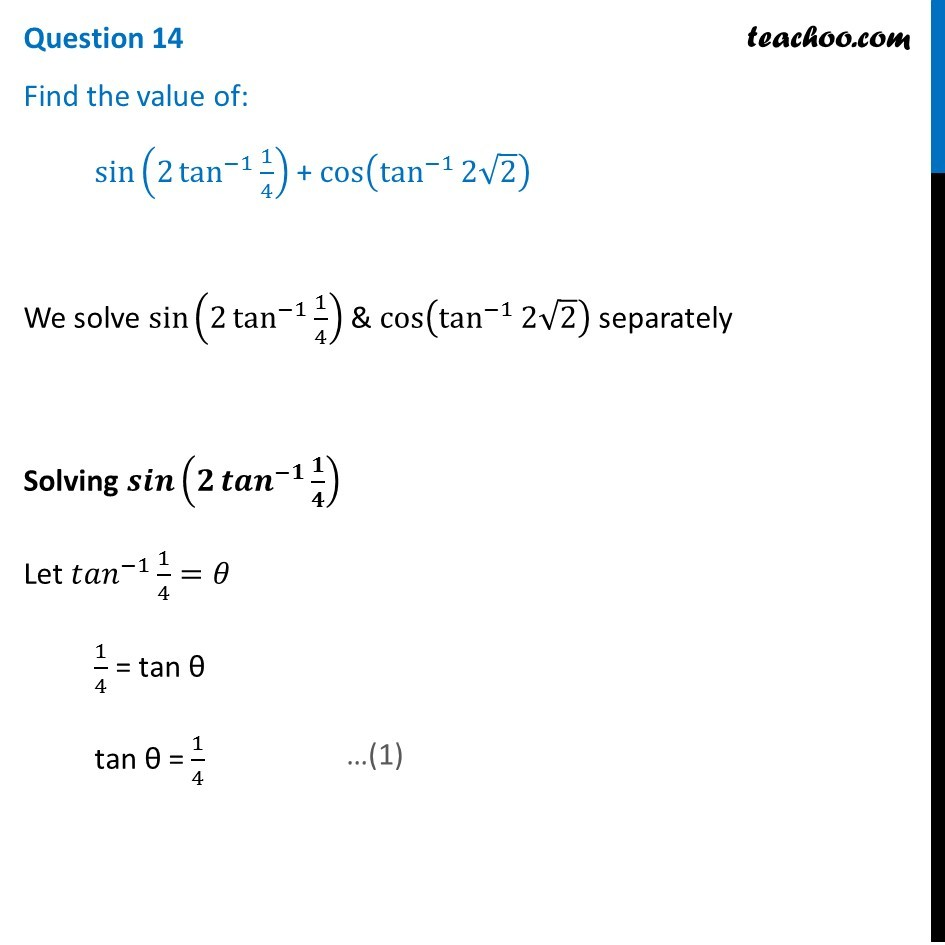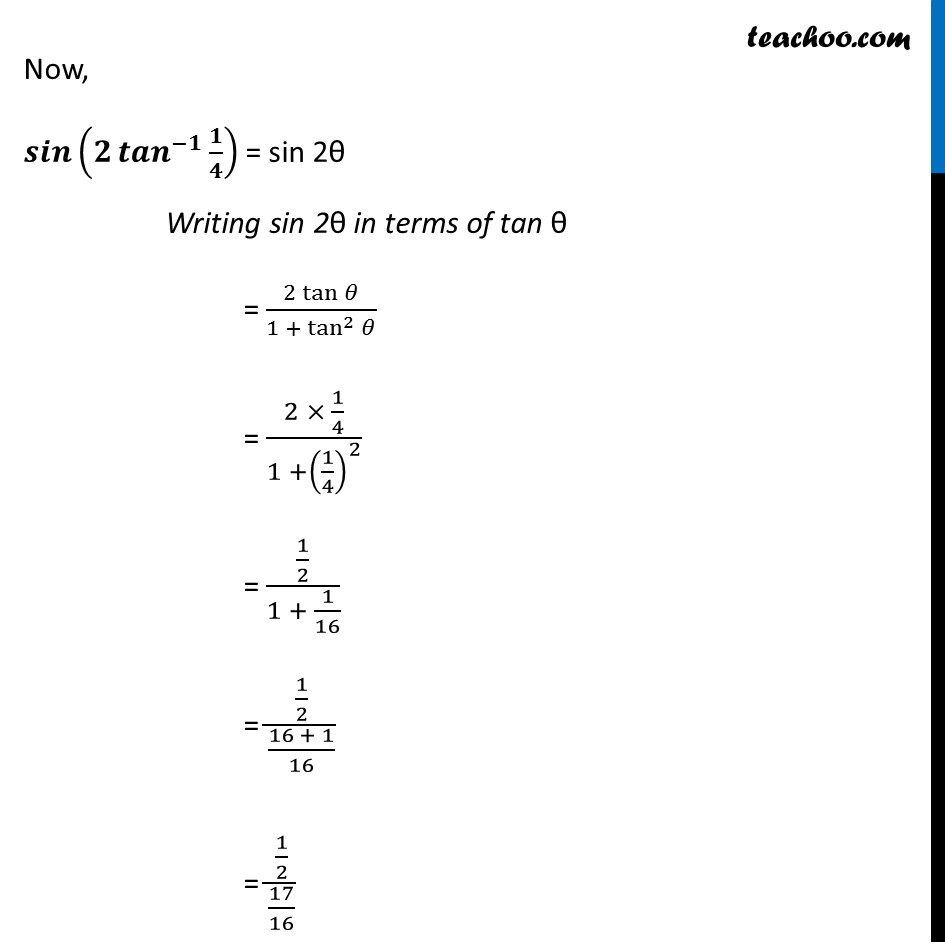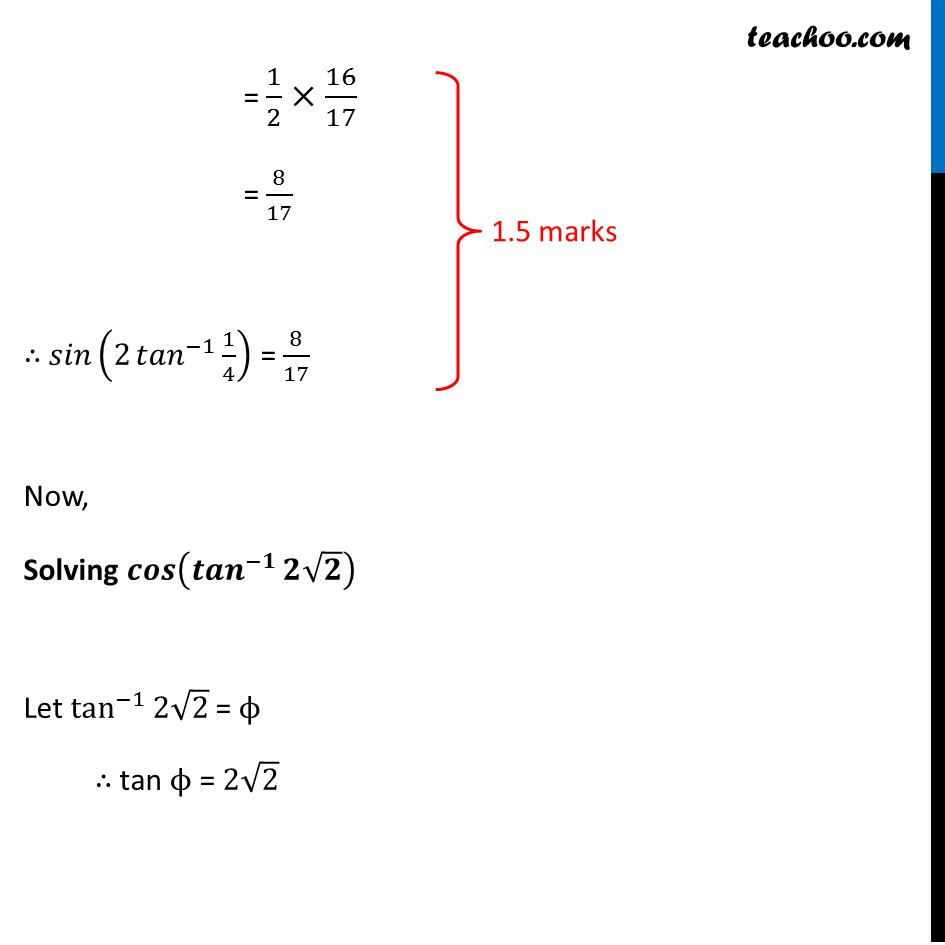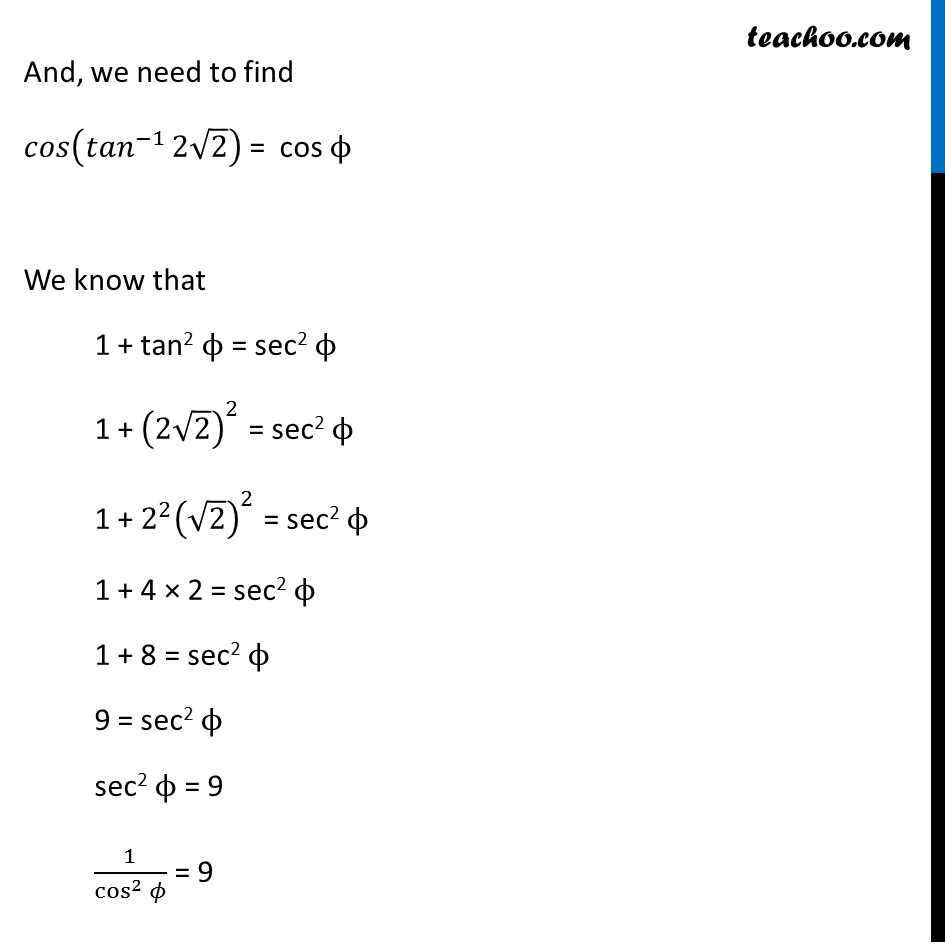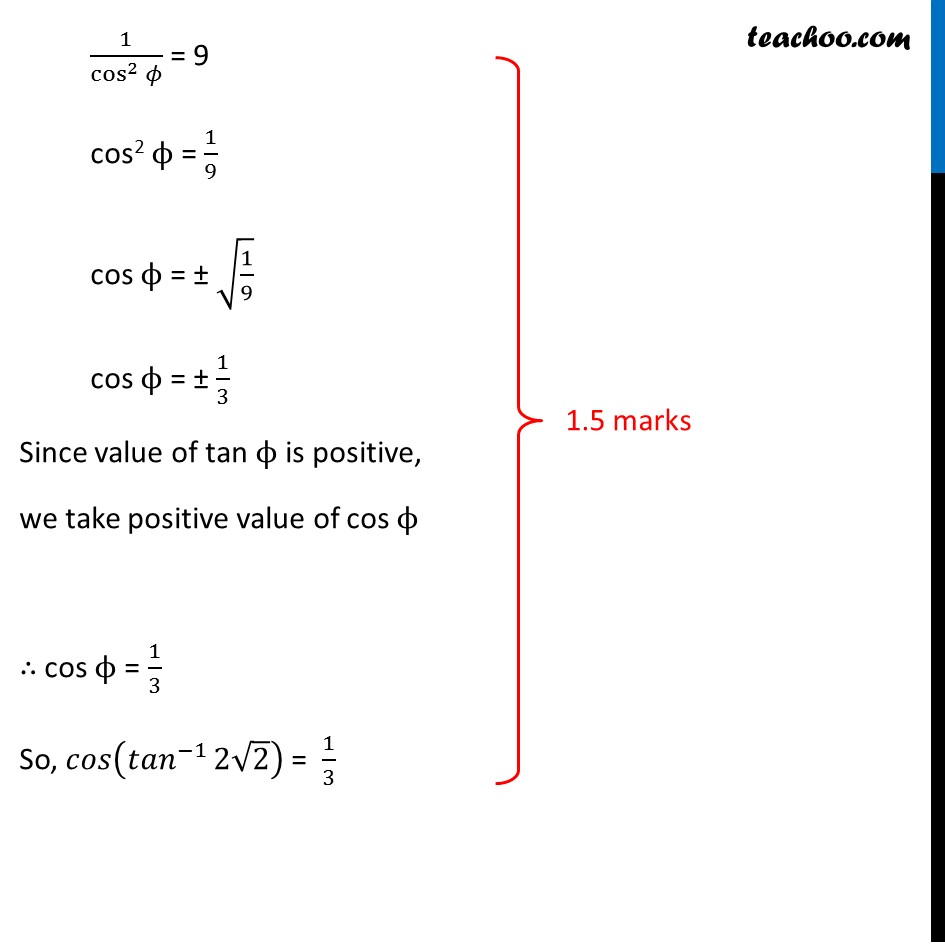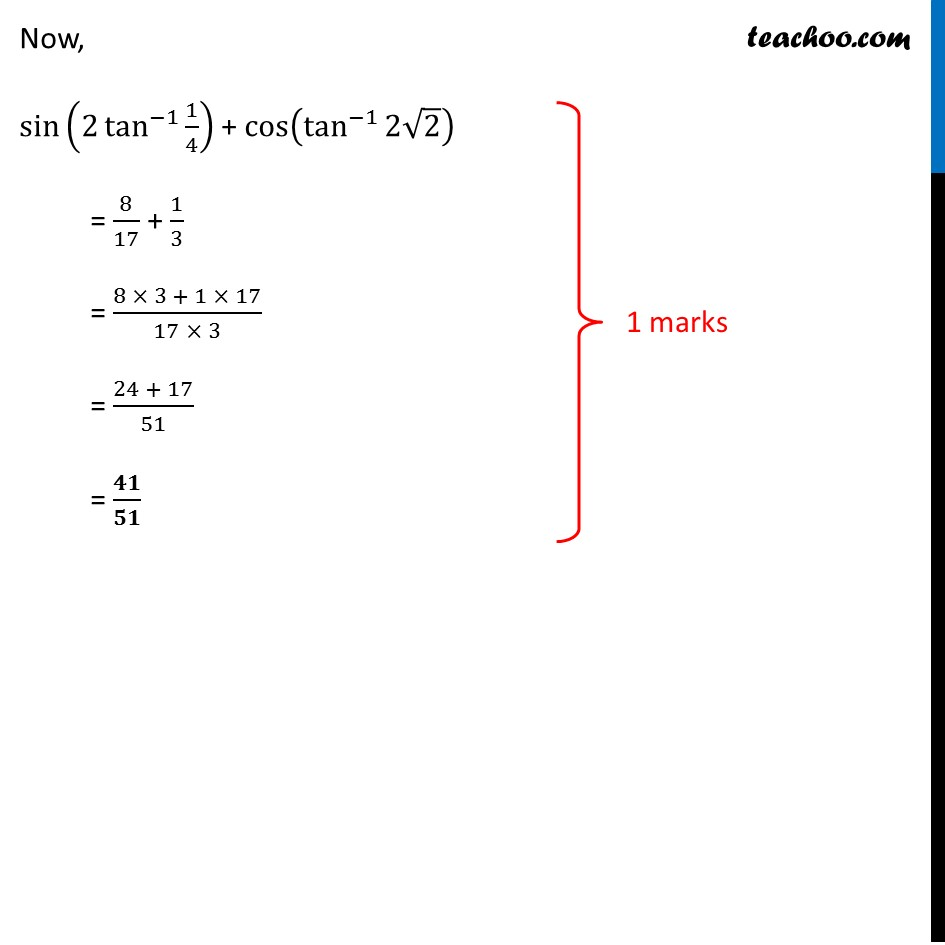Learn in your speed, with individual attention - Teachoo Maths 1-on-1 Class

### Transcript

Question 14 Find the value of: sin⁡(2 tan^(−1)⁡〖1/4〗 ) + cos⁡(tan^(−1)⁡〖2√2〗 ) We solve sin⁡(2 tan^(−1)⁡〖1/4〗 ) & cos⁡(tan^(−1)⁡〖2√2〗 ) separately Solving 𝒔𝒊𝒏⁡(𝟐 〖𝒕𝒂𝒏〗^(−𝟏)⁡〖𝟏/𝟒〗 ) Let 〖𝑡𝑎𝑛〗^(−1)⁡〖1/4〗=𝜃 1/4 = tan θ tan θ = 1/4 Now, 𝒔𝒊𝒏⁡(𝟐 〖𝒕𝒂𝒏〗^(−𝟏)⁡〖𝟏/𝟒〗 ) = sin 2θ Writing sin 2θ in terms of tan θ = (2 tan⁡𝜃)/(1 + tan^2⁡𝜃 ) = (2 × 1/4)/(1 +(1/4)^2 ) = (1/2)/(1 + 1/16) = (1/2)/((16 + 1)/16) = (1/2)/(17/16) = 1/2×16/17 = 8/17 ∴ 𝑠𝑖𝑛⁡(2 〖𝑡𝑎𝑛〗^(−1)⁡〖1/4〗 ) = 8/17 Now, Solving 𝒄𝒐𝒔⁡(〖𝒕𝒂𝒏〗^(−𝟏)⁡〖𝟐√𝟐〗 ) Let tan^(−1)⁡〖2√2〗 = ϕ ∴ tan ϕ = 2√2 And, we need to find 𝑐𝑜𝑠⁡(〖𝑡𝑎𝑛〗^(−1)⁡〖2√2〗 ) = cos ϕ We know that 1 + tan2 ϕ = sec2 ϕ 1 + (2√2)^2 = sec2 ϕ 1 + 〖2^2 (√2)〗^2 = sec2 ϕ 1 + 4 × 2 = sec2 ϕ 1 + 8 = sec2 ϕ 9 = sec2 ϕ sec2 ϕ = 9 1/cos^2⁡𝜙 = 9 And, we need to find 𝑐𝑜𝑠⁡(〖𝑡𝑎𝑛〗^(−1)⁡〖2√2〗 ) = cos ϕ We know that 1 + tan2 ϕ = sec2 ϕ 1 + (2√2)^2 = sec2 ϕ 1 + 〖2^2 (√2)〗^2 = sec2 ϕ 1 + 4 × 2 = sec2 ϕ 1 + 8 = sec2 ϕ 9 = sec2 ϕ sec2 ϕ = 9 1/cos^2⁡𝜙 = 9 1/cos^2⁡𝜙 = 9 cos2 ϕ = 1/9 cos ϕ = ± √(1/9) cos ϕ = ± 1/3 Since value of tan ϕ is positive, we take positive value of cos ϕ ∴ cos ϕ = 1/3 So, 𝑐𝑜𝑠⁡(〖𝑡𝑎𝑛〗^(−1)⁡〖2√2〗 ) = 1/3 Now, sin⁡(2 tan^(−1)⁡〖1/4〗 ) + cos⁡(tan^(−1)⁡〖2√2〗 ) = 8/17 + 1/3 = (8 × 3 + 1 × 17)/(17 × 3) = (24 + 17)/51 = 𝟒𝟏/𝟓𝟏